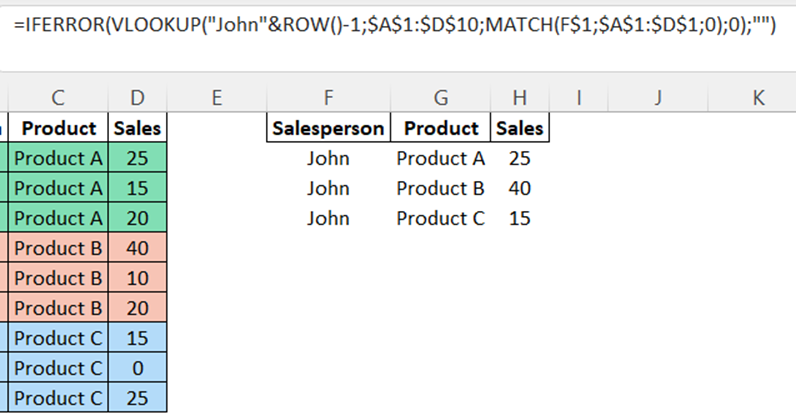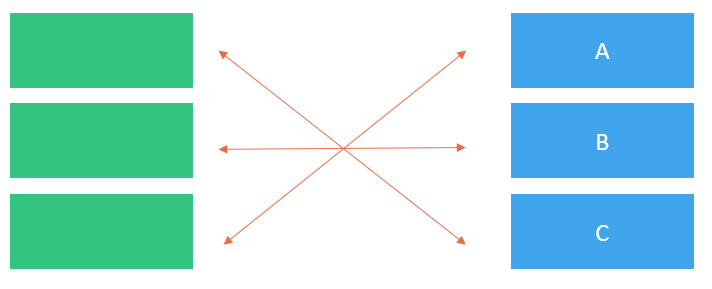top of page

# Excel lookup functions with multiple criteria or multiple results

Updated: Jun 12

As an Excel user, you're likely familiar with the lookup functions like VLOOKUP, INDEX/MATCH and XLOOKUP and their ability to retrieve a single value based on a criterion.

But what if you have multiple criteria and you want to return one result or you have one criterion and you want to get multiple results?Multiple criteria or multiple results

In this post, we'll see some advanced lookup functions and the FILTER function to help you look up in Excel in a smart way.

TABLE OF CONTENT

## Look Up with Multiple Criteria

### Using VLOOKUP with a Helper Column

Suppose you have a table of sales data with the 3 following columns: Product, Salesperson, and Sales. You want to retrieve the sales value for a specific product ("Product A") and a salesperson ("Henry").Sales table

First, you need to create a new column that concatenates the first two columns using the following formula:

`=A2&B2 or =CONCATENATE(A2;B2)`Helper Column

You can now use a VLOOKUP function with the concatenation of your 2 criteria with:

`=VLOOKUP("Product A"&"Henry";C2:D10;2;0)`

In this example, the formula concatenates the two joined criteria using the "&" operator.

The range C2:D10 represents the table range, and the number 2 indicates that the sales value is in the second left-most column.VLOOKUP - Multiple Criteria

By the way, if you want to know how to use the Double or Nested VLOOKUP that runs way faster than the standard VLOOKUP, here is the post you need.

### Using XLOOKUP

One easy way of performing a multiple criteria lookup is by using XLOOKUP with the "&" operator that concatenates all the criteria into one lookup value and their corresponding lookup columns into one lookup array.XLOOKUP and CONCATENATE

This approach is more efficient than using VLOOKUP because you don't need the helper column.

### Using the FILTER function

If your version of Excel has dynamic arrays (Office 365), you can use the new FILTER function to return the results that you need.FILTER function using multiple criteria

This formula filters the range C2:C10 based on the two following criteria: Product A and Henry.

The (A2:A10="Product A")*(B2:B10="Henry") part creates an array of TRUE and FALSE values, where TRUE represents the rows that match both criteria.

The FILTER function then returns the corresponding prices from the range C2:C10 for the matching rows.

Now, you know how to retrieve a value with multiple criteria, but what if you want to get multiple matches based on one criterion?

## Look up and Returning Multiple Results

### Using VLOOKUP, a Helper Column and ROW

By default, VLOOKUP returns the first match it finds but by combining this function with ROW, an array function, you can get multiple matches.

First, you need to add a helper column that concatenates the values you are looking up (the salesperson names in column B here) with a unique number using the following formula:

`= B2&COUNTIF(\$B\$2:B2;B2)`Helper column

Then, you need to write the fields you want to retrieve from the dataset (i.e.: Salesperson, Product and Sales from F1 to H1).

Finally, if you are interested in getting the information regarding John’s sales, here is the VLOOKUP function you should write in F2 and then paste in the cells below the 3 fields.

`=VLOOKUP(“John”&ROW()-1;\$A\$1:\$D\$9;MATCH(F\$1;\$A\$1:\$D\$1;0);0)`VLOOKUP with multiple results

"John"&ROW()-1 will replicate the Salesperson index created in the Helper column and the MATCH function helps you target the array you are interested in.

To remove all the N/A errors, you can use the IFERROR function (see the screenshot below):Clean VLOOKUP with multiple results

Unlikely VLOOKUP, XLOOKUP can't retrieve multiple matches but here is a powerful way to do so.

### Using the FILTER function

An efficient approach is to use the FILTER function as you will only need to specify the criteria you want (here, we want to retrieve from the dataset the lines matching only John in the column B) and you will get all the results found for this criteria.FILTER function to get multiple results

## Look up with Multiple Criteria and Return Multiple Results

After looking up with different criteria and returning multiple results based on 1 criterion, let's see how you can get multiple results based on multiple criteria.Multiple Criteria and Return Multiple Results

### Using the FILTER function

As the FILTER function only provides one argument called "Include" to target data, the trick to handle a many-to-many relationship is to create a logical expression that uses Boolean algebra to target the data of interest and supply this expression as the include argument.

In this example, I want to retrieve from a table with 2 columns (Names and Values) the values matching the names John and Jack.

Prior to using the following formula, indicate in F3 and F4 the criteria John and Jack:

`=FILTER(B3:C11;ISNUMBER(MATCH(B3:B11;F3:F4;0)))`Multiple criteria and multiple results

The first argument is the lookup range.

The second argument can be split into 2 parts.

`=ISNUMBER(MATCH(B3:B11;F3:F4;0))`

This MATCH function creates a number series based on the 2 criteria.

The ISNUMBER function creates a Boolean array of TRUE and FALSE.Boolean table

Almost everybody using Excel knows VLOOKUP and XLOOKUP, but few people know how to combine them with some other functions like CONCATENATES, ROW or how to look up values with FILTER.

Now that you know, you can tackle more complex data analysis tasks and level up your efficiency in Excel.

If you are an heavy user of Excel, you will most certainly like the Power-user add-in. Get dozens of new advanced features that will make you the boss of Excel!

413 views

See All
bottom of page